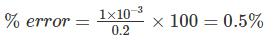Courses

# Test: Electrical And Electronic Measurements- 5

## 10 Questions MCQ Test Mock Test Series for Electrical Engineering (EE) GATE 2020 | Test: Electrical And Electronic Measurements- 5

Description
This mock test of Test: Electrical And Electronic Measurements- 5 for Electrical Engineering (EE) helps you for every Electrical Engineering (EE) entrance exam. This contains 10 Multiple Choice Questions for Electrical Engineering (EE) Test: Electrical And Electronic Measurements- 5 (mcq) to study with solutions a complete question bank. The solved questions answers in this Test: Electrical And Electronic Measurements- 5 quiz give you a good mix of easy questions and tough questions. Electrical Engineering (EE) students definitely take this Test: Electrical And Electronic Measurements- 5 exercise for a better result in the exam. You can find other Test: Electrical And Electronic Measurements- 5 extra questions, long questions & short questions for Electrical Engineering (EE) on EduRev as well by searching above.
QUESTION: 1

### For the bridge shown Z1 = 200 ∠20° Ω, Z2 = 150 ∠30° Ω  and Z3 = 300 ∠-30° Ω. What is the value of Z4 so that the bridge is balanced?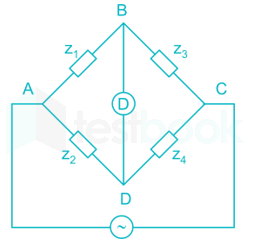Solution:

At bridge balance condition,
Z1 Z= Z2 Z3
⇒ 200 ∠20° Z4 = 150 ∠30°    300 ∠-30°
⇒ Z4 = 225 ∠-20° Ω

*Answer can only contain numeric values
QUESTION: 2

### A simple slide wire potentiometer is used for measurement of current in the circuit. The voltage drop across a standard resistor of 0.6Ω is balanced at 60 cm. The magnitude of current if the standard cell of 4V is balanced at 50 cm is ______ (in A)

Solution:

Standard cell of 4 V is balanced at 50 cm.
The voltage drop across 60 cm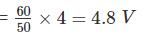The working current through the circuit is,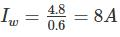QUESTION: 3

### In the bridge circuit shown in figure when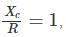then the voltmeter reads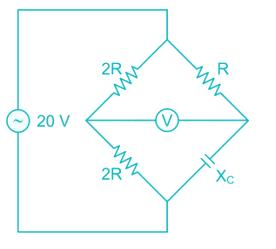Solution:

At bridge balance condition
(2R) (Xc) = (2R) (R)
Given that,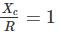at this condition, bridge is balanced
Hence the voltmeter reading is 0 V.

*Answer can only contain numeric values
QUESTION: 4

A resistance potentiometer has a total resistance of 4 kΩ and is rated 10W. If the range of potentiometer is 0 to 200 mm. then its sensitivity is _______ (in V/mm)

Solution:

R = 4 kΩ
P = 10 W
We know that.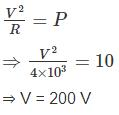The potentiometer range is 0 to 200 mm.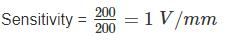QUESTION: 5

A length of cable was tested for insulation resistance using loss of charge method. A capacitance formed by sheath of cable of 300 PF is found to have drop in voltage from 300 V to 100 V in 120 seconds. Calculate the insulation resistance of the cable in MΩ

Solution:

Given that = C = 300 pF
V1 = 300 V
V2 = 100 V
t = 120 S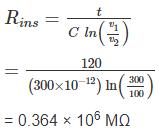*Answer can only contain numeric values
QUESTION: 6

Calculate the value of effective resistance (in Ω) at a supply frequency of 100 Hz. (C= 2 μF)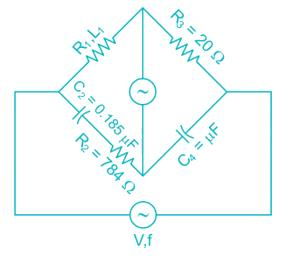Solution:

Under balance condition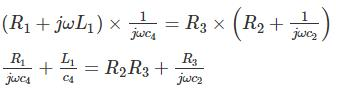Separate and equating real and imaginary part.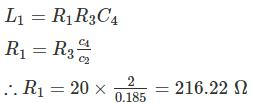*Answer can only contain numeric values
QUESTION: 7

Find the excitation frequency (in Hz) in the Ac Bridge shown in figure under balance condition. The circuit component values are given as
R1 = 100 kΩ,
R3 = R4 = 100 kΩ,
C1 = 2 C2 = 10 nF

Solution:

under bridge balance condition,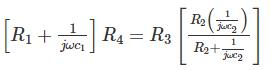Given that, R3 = R4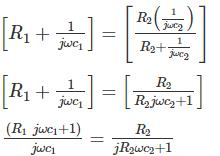(1 + jωR1C1)(1 + jωR2C2) = jωC1R2
1 + jωR1C+ jωR2C− ω2R1R2C1C= jωC1R2
By comparing real parts on both sides,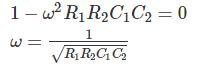By comparing imaginary parts on both sides,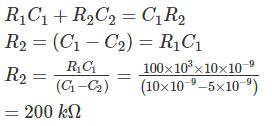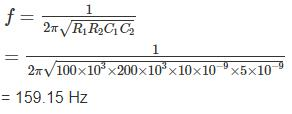QUESTION: 8

The Schering bridge shown in figure has the following constant R1 = 1.5 kΩ, C1 = 0.4 μF, R2 = 3 kΩ and C3 = 0.4 μF at frequency 1 kHz. The dissipation factor is_______

Solution:

Given, R1 = 1.5 kΩ
C1 = 0.4 μF
R2 = 3 kΩ
C3 = 0.4 μF
f = 1 kHz
We know that,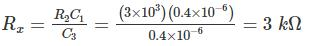and the unknown capacitance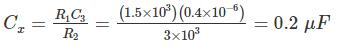Dissipation factor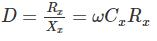= 2πfCxRx
= 2π × 1 × 103 × 0.2 × 10-6 × 3 × 103
= 3.77

*Answer can only contain numeric values
QUESTION: 9

The AC bridge is supplied with a source of frequency 5 kHz as shown in the figure. If the bridge is balanced at R1 = 4 R2 and C3 = 20 μF, the value of unknown capacitor Cx is _____ (in μF)

Solution:

At bridge balanced condition,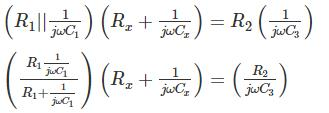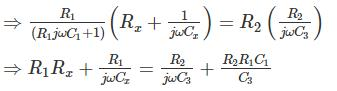By comparing imaginary part,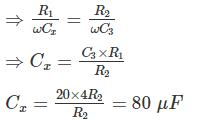QUESTION: 10

The dc potentiometer shown in the figure has working current of 10 mA with switch S open. Let Rg + R1 = 100Ω. The galvanometer G can only detect currents greater than 10 μA. The maximum percentage error in the measurement of the unknown e.m.f. Ex as calculated from the slider position shown is closest to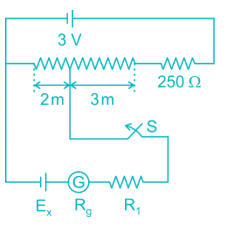Solution:

Given that, when the switch is open.
IW = 10 mA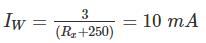⇒ Rx = 50 Ω.
Length of Rx = 5m.
Resistance across 3m = 30 Ω
Resistance across 2m = 20 Ω
Voltage across 20 Ω = 20 × 10 × 10-3 = 0.2 V
Errors in the reading are due to Rg and R1.
Error = voltage drop across Rg and R1
= Ig (Rg + R1)
= 10 × 10-6 (100) = 1 mV